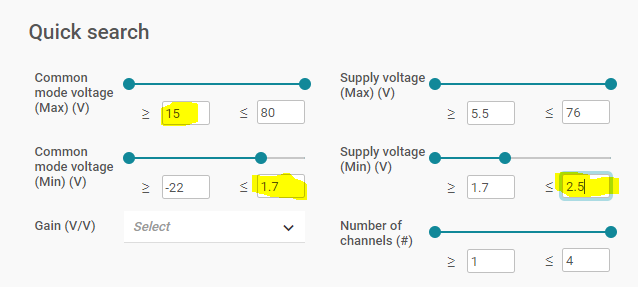If you have a related question, please click the "Ask a related question" button in the top right corner. The newly created question will be automatically linked to this question.

# INA139: What is range of current that can be measured

Part Number: INA139
Other Parts Discussed in Thread: INA190, INA191, INA186

Hi,

I have referred to the datasheet and found that there is a VOUTMAX which indirectly imposes a limitation on current measurement.

My application would be to have this on different VRM's ranging from 0.1mA to 10A. Could you give me the Rs and RL values for following conditions with which the circuit will have the best efficiency

V+  = 2.5v

1. Vin+ = 1.8v, expected current drawn = 0.1mA to 1A

2. Vin+ = 15v, expected current drawn = 0.1mA to 1A

Thanks,

Murali

• Hello Murali,

Thanks for using the forum for you question.  First I do not think the INA139 will work in your application.  V+ is limited to 2.7 to 40V and is not specified at 2.5V.  Also in condition #1 the common mode is limited to the same range of 2.7V to 40V.

Have you attempted to use our parametric search to limit the options of Current Sense Amplifiers?

I would start here.  http://www.ti.com/amplifier-circuit/current-sense/overview.html

And if you would like an analog input click on the link for that as you can see there are other options.  I did a search for your conditions for both cases and here is the link for analog output.  Below are the parameters I changed.  It looks like maybe the INA186, INA190 and INA191 could be options for you.• Hi Javier,

INA186 datasheet says the following "The INA186 is a specially designed, current-sensing amplifier that accurately measures voltages developed across current-sensing resistors on common-mode voltages that far exceed the supply voltage. Current is measured on input voltage rails as high as 40 V at VIN+ and VIN– , with a supply voltage, VS, as low as 1.7 V."

In most of my cases, common mode voltage is in between 0.6v and 3v and the supply voltage is either of 2.5v, 3.3v or 5v.  INA186 says that it measures accurately when there is a considerable difference between common mode voltage and supply voltage.

Thanks,

Murali

• Hello Murali,

Most of our current sense amplifiers have ability to have the input common mode voltage exceed the voltage supply. That is what the statement refers too.
So for your conditions you can have a common mode voltage at 3V even though your VS supply is at 2.5V. Please be aware that your output voltage will be limited based on your supply voltage. So you may need to change the selection of your INA186 gain or Rshunt resistor for the variation of supply voltage.
• Hi Javier,

I would like to know if there is any minimum requirement on the Rsense resistor value. I see there is a max limit value on Rsense as 1kohm.

Thanks,
Murali
• Hello Murali,

There is no requirement for low value but you need to make sure that you can measure it accurately.  For example if you have a large current  ( Hundreds of Amps) and require µΩ resistors you then need to take into account the resistance of the solder connection to your resistors.  At 1 A that is less of an issue as you will most likely use a larger resistor where the solder resistance is not an issue.

• Hi Javier,

Following are my design parameters,
High-side configuration, Vs = 3.3v, Vref = 0v, Gain = 25, and expected current drawn is in in the range of 1mA to 2A.

I have calculated the Rsense max using the equation Rsense < (Vsp)/(Imax x Gain) and the obtained value is 0.0652ohms, where Vsp = Vs-40mV

After I have calculated the Rsense, I have used the Vout = Imin x Rsense x Gain equation to see if my Vout is in the output voltage swing limits.

Iload(A) Rsense(ohm) GAIN Vref(volt) Vout from the equations (Volt)
2 0.06 25 0 3
1 0.06 25 0 1.5
0.1 0.06 25 0 0.15
0.01 0.06 25 0 0.015
0.001 0.06 25 0 0.0015

1. Vout column has the voltages within the limits (greater than gnd+1mV and less than Vs-40mv)
2. I haven't included the Common mode voltage into my calculations. Is this the right way of doing the calculations? I only took into consideration while calculating the values
3. I see the resistor value is only 0.06ohm. Is that a problem?
4. What sort of probes do you recommend to measure 0.0015v Vout?

Thanks,
Murali
• Hello Muralidhar,

The issue you will come across is that your VOS for the INA186 is 50uV and your swing to GND will be limited to 1mV.   We have a detailed video series that can help you with many of your questions.

Your measurement will be dependent of your instrument.  The specification will be on the meter or ADC you are using.